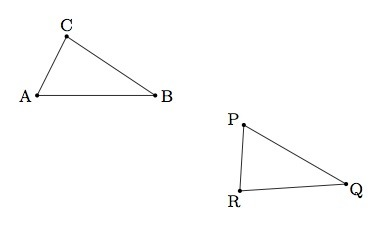# Showing a triangle congruence: the general case

Alignments to Content Standards: G-CO.A.5

Suppose $\triangle ABC$ and $\triangle PQR$ are distinct, congruent triangles. Using the steps below, show that a congruence can always be shown with one, two, or three rigid motions:

1. A translation taking $A$ to $P$ (if necessary).
2. A rotation taking $B$, or the image of $B$ after translation, to $Q$ (if necessary).
3. A reflection about $\overleftrightarrow{PQ}$ which takes $C$, or its image after (a) and (b), to $R$ (if necessary).

## IM Commentary

The purpose of this task is to work with transformations to exhibit triangle congruences in a general setting. The specific steps indicated here are not the only way to show a triangle congruence and the teacher may wish to have students create their own sequence of transformations. The method used here has the advantage that each of the basic rigid motions (translations, rotations, and reflections) is used. Students may struggle with the general setting here. No pictures have been provided in the problem because its goal is to set forward a sequence of steps that will work regardless of which two congruent triangles are given. The teacher should encourage students to draw their own examples and then work in groups to compare and discuss their findings. Ideally, some students will choose examples where one or more of the three steps is not necessary so that the students have an opportunity to see why the ''if necessary'' clauses are added in all three steps. For example, if the points $A$ and $P$ happen to coincide, then the first step translating $A$ to $P$ is not needed.

For additional work with rigid motions, the teacher may wish to ask students to give examples for different scenarios. In particular, students can provide an example where only steps (a) and (c) are needed to show the congruence, or an example where only steps (a) and (b) are required. There are many possible combinations and this will help build visual intuition for the impact of the different rigid motions.

This task provides an ideal setting to engage in MP2, Reason Abstractly and Quantitatively. The central difficulty with this problem is trying to understand how the abstract method described in this problem applies in many different concrete cases. Therefore students should be thinking both about the abstract sequence of steps and how these steps would apply in multiple settings. The task also encourages development of MP5, Use Appropriate Tools Strategically. In order to show the different rigid motions, students can use computer software, paddy paper, or transparencies.

## Solution

For our solution, we follow through an example of two triangles $ABC$ and $PQR$ where all three steps are needed and show representative pictures. For a different choice of triangles $ABC$ and $PQR$, some of the steps may not be necessary. Our sample triangles are pictured below:1. Since $A \neq P$ we need a translation to move $A$ to $P$ and this is pictured below:The image of $\triangle ABC$ after the translation is denoted $\triangle A^\prime B^\prime C^\prime$.

2. Since $B^\prime \neq Q$ in our example, we use a rotation to send $B^\prime$ to $Q$. We have already arranged for $A^\prime = P$ so we take $P$ as our center of rotation and to send $B^\prime$ to $P$ we need to rotate by angle $B^\prime PQ$. This is pictured below:3. In our case, $C^{\prime \prime} \neq R$ so our last step is to reflect about $\overleftrightarrow{PQ}$. If we call $S$ the image of $C^{\prime \prime}$ under this reflection, we claim that $S= R$ and so our congruence is complete. The reason why $S = R$ is that $\triangle PQR$ and $PQS$ share side $\overline{PQ}$. Moreover $m(\angle PQR) = m(\angle PQS)$ and $m(\angle QPR) = m(\angle QPS)$ by the original hypothesis that $\triangle ABC$ is congruent to $\triangle PQR$. Thus $R = S$.